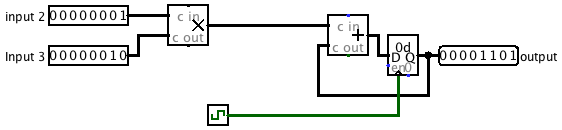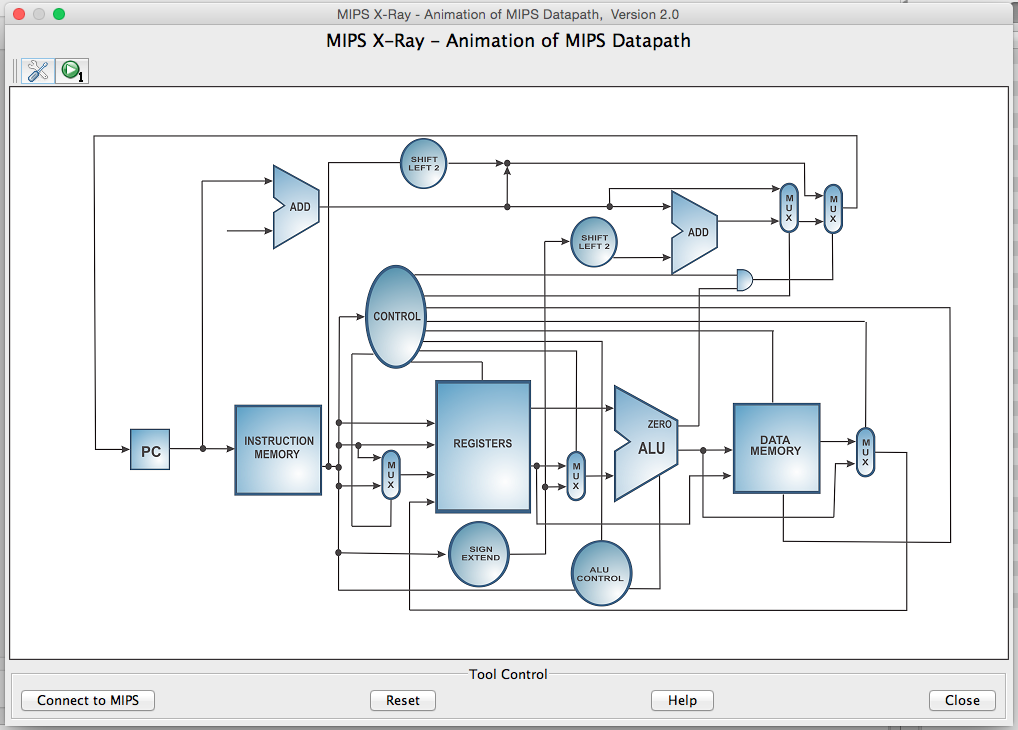## Lab 7

Computer Architecture I ShanghaiTech University
Lab 6 Lab 7 Lab 8

## Exercises

### Exercise 1: Fibonacci Numbers

So far we have built circuits that are either 1) purely combinational and require no clock or 2) have memory but run infinitely. In this exercise, we want to build a circuit that will compute the Nth Fibonacci number. As a quick review the Fibonacci numbers are defined by F0 = 0, F1 = 1, and Fi = Fi-1 + Fi-2.

1. Start with just the infinite Fibonacci computation. Hopefully this should be pretty intuitive based on previous exercises that you have done before. How many registers do you need? What arithmetic blocks do you need? Where should the output be attached? Make sure you figure out a way to properly initialize the registers for the computation to work.
2. Your sub-circuit will compute up to the 15th Fibonacci number and we will assume our input N > 0 is an unsigned number. How many input bits do you need and how many output bits do you need to represent the 15th Fibonacci number?
3. Now to prevent the circuit from computing the Fibonacci numbers to infinity, we will make use of the Enable bit (lower left) on registers. When unset or pulled high, the registers will continue to load their inputs on the rising edge of the CLK. If Enabled is pulled low, then the registers will NOT load their inputs. We need to create a signal that will properly go low when we want the circuit to stop computing Fibonacci numbers.
4. Create an additional part of the circuit that halts the original circuit after N computations. For this exercise you may find the following blocks useful: Counter (Memory), Comparator (Arithmetic), and Probe (Wiring). Make sure that it properly stops on the Nth Fibonacci number (watch for off-by-one errors) and that it actually stays there (run it for at least 20 clock cycles).
Your output should be in binary, but you can use a probe to display the value in decimal. Make sure you check the attributes of any counter and comparator you use.

Bonus: With proper register initialization, you can get this circuit fib15 to work properly for N > 0. But register initialization is annoying and must be repeated every time you reset your circuit. With a clever addition, you can get this fib15 to work for N >= 0 without the need for register initialization (Hint: it involves a MUX).

#### Checkoff

• Show your TA your fib15 circuit and verify that for N = 15 after some time it will return Out = 610 indefinitely.

## Exercise 2: Pipelining

This next exercise will get you some hands-on practice with pipelining. Assume that on power-on, registers initially contain zeros.

Consider the following 2-input FSM. Its next state and output is computed by multiplying the inputs and adding it to the current state.Say the propogation delay of a adder block is 50ns, the propogation delay of a multiplication block is 55 ns, and the clk-to-q delay of a register is 5ns. Calculate the maximum clock rate at which this circuit can operate. Assume that the register setup time is negligible, and that both inputs come from clocked registers that receive their data from an outside source.

#### Checkoff

• Show your TA the calculations you performed to find the maximum clock rate (non-pipelined).

We want to improve the performance of this circuit, and let it operate at a higher clock rate. To do so, we will divide up the multiplication and addition into two different pipeline stages; in the first pipeline stage, we will perform the multiplication of the two inputs. In the second pipeline stage, we will add the product to the state.

Our definition of "correctness" will be simple: we will consider the sequence of outputs from this circuit "correct" iff it corresponds to the sequence of outputs the non-pipelined version would emit, potentially with some leading zeros. For example, if for some sequence of inputs the non-pipelined version emits [3,5,1,2,4, ...], a correct circuit might emit the sequence of outputs [0,3,5,1,2,4, ...] for that same sequence of inputs.

For your convenience and to help standardize check-offs, we are providing a starting point in the files pipeline.circ and ROMdata. In pipeline.circ, the sub-circuit Non-pipelined is set up exactly as the figure above. The main circuit is set up to produce the output sequence [3,5,1,2,4,-1,0,0,...] on the non-pipelined version of this circuit. It is also a handy example of how to use memory from a file. The ROM block should be initialized to the proper data, but if it is zero-ed out, right-click it and choose "Load image..." and select ROMdata.

Note that we need a register to hold the intermediate value of the computation between pipeline stages. This is a general theme with pipelines.

1. Complete the sub-circuit Pipelined. You will need to add a register to divide the multiplication and addition into separate pipeline stages.
2. Calculate the maximum clock rate for the pipelined version of the circuit.
3. When we talked about pipelining in lecture, we discussed that if a computation depends on the output of a previous computation, it's difficult to pipeline them and we often need to insert a pipeline "bubble" (or several) to ensure that the output of the first computation is ready to be an input to the second. Explain why inserting such "bubbles" is unnecessary for this particular circuit.

#### Checkoff

• Show your TA the completed, pipelined circuit.
• Show your TA the calculations you performed to find the maximum clock rate (pipelined).
• Explain to your TA why bubbles are unecessary in this circuit.

## Exercise 3: Datapath

It turns out that MARS 4.5 has a new feature called MIPS X-RAY that will visualize the datapath while you are running your instruction. This might be useful for you for project 2!
To open up this feature go under "Tools" and select "MIPS X RAY". It should open a window that looks like this:We will use the file fibonacci.s to see how each instruction goes through the datapath. Open and the MIPS X-RAY feature. You can connect the X-RAY to the instructions by pressing the "Connect to MIPS" button on the bottom left of the screen. Assemble the file by pressing the "Assemble" button on the upper left of the screen. You can then step through the code using the green "Step" button next to the "Assemble" button. The wires that are red signal that the control signal is on. You can also click on any control block to see what is going on inside of it. Play around with the feature, and the following questions will be based off what is going on in the control block.

Note: The X-RAY feature generalizes instructions to have the same opcode. You can see this in control with the instructions add and addi. For the questions below, base your soley on the logic of the control block.

Questions:

• What do the bits BIT 0 - BIT 5 represent?
• For what instruction or type of instructions is opALU0 active? What operation in the ALU does opALU0 represent?
• For what instruction or type of instructions is opALU1 active?
• For what instruction or type of instructions is ALUSrc active? What does this signal represent with respect to the input to the ALU?